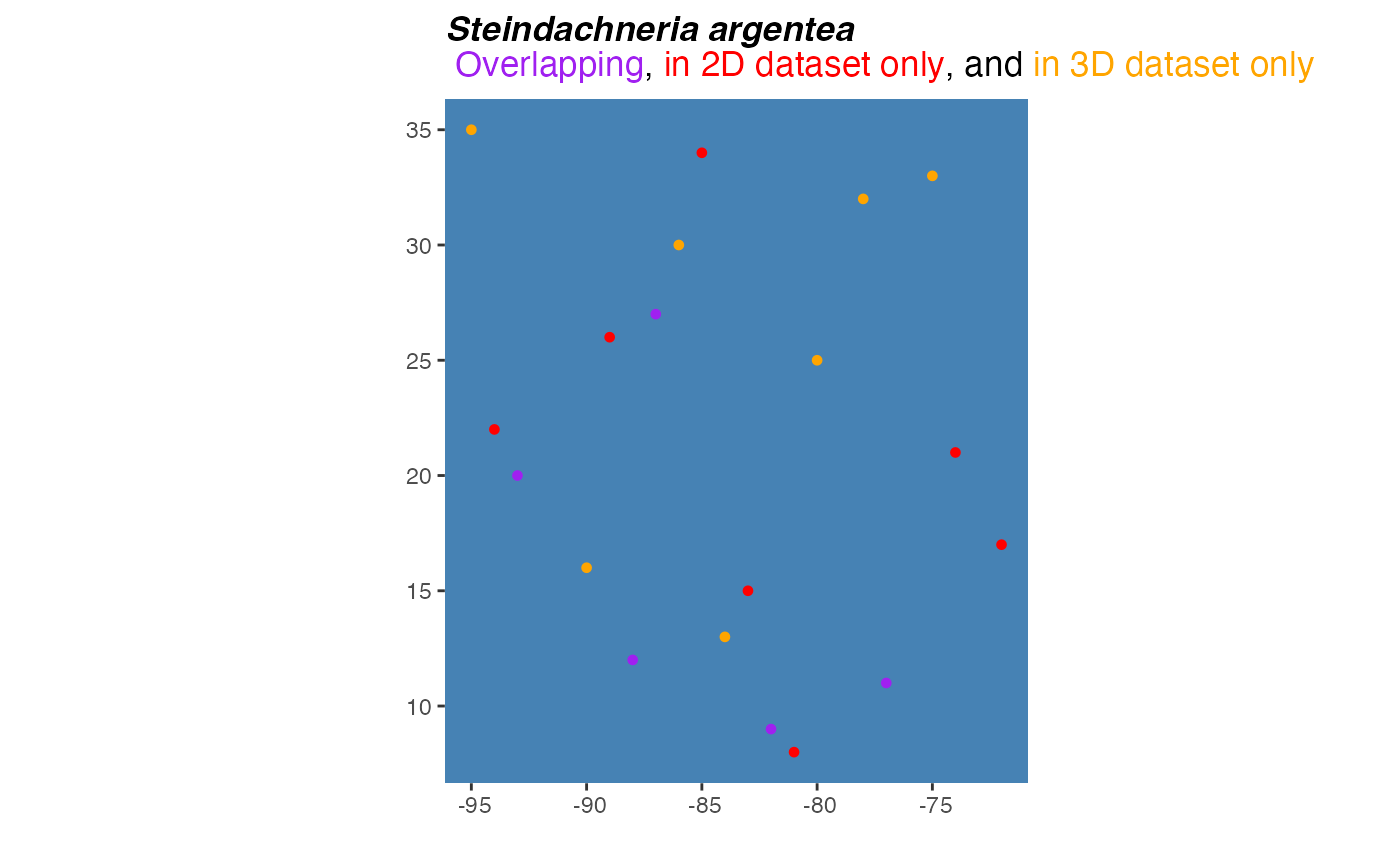A convenient wrapper around ggplot to generate formatted plots comparing two sets of occurrence point plots.

pointCompMap(
occs1,
occs2,
spName,
land = NA,
occs1Col = "#bd0026",
occs2Col = "#fd8d3c",
agreeCol = "black",
occs1Name = "Set 1",
occs2Name = "Set 2",
landCol = "gray",
waterCol = "steelblue",
ptSize = 1,
verbose = TRUE,
...
)

## Arguments

occs1

A data.frame with at least two columns named "longitude" and "latitude" or that can be coerced into this format.

occs2

A data.frame with at least two columns named "longitude" and "latitude" or that can be coerced into this format.

spName

A character string with the species name to be used in the plot title.

land

An optional coastline polygon shapefile of types sf or SpatRaster to provide geographic context for the occurrence points.

occs1Col

Color for occurrence points on map

occs2Col

Color for occurrence points on map

agreeCol

Color for occurrence points shared between occs1 and occs2.

occs1Name

An optional name for the first set of occurrences, which will be color-coded to occs1Col in the resulting plot.

occs2Name

An optional name for the first set of occurrences, which will be color-coded to occs2Col in the resulting plot.

landCol

Color for land on map

waterCol

Color for water on map

ptSize

numeric value for cex; size of occurrence points on map.

verbose

logical. Switching to FALSE mutes message describing which columns in occs1 and occs2 are interpreted as x and y coordinates.

...

Additional optional arguments to pass to ggplot initial plot object.

## Value

A ggplot plot object.

## Note

The x and y column names of occs1 and occs2 must match.

ggplot

## Examples

set.seed(5)
occs <- data.frame(cbind(decimalLatitude = sample(seq(7,35), 24),
decimalLongitude = sample(seq(-97, -70), 24)))

set.seed(0)
occs1 <- occs[sample(1:nrow(occs),
size = 12, replace = FALSE),]
set.seed(10)
occs2 <- occs[sample(1:nrow(occs),
size = 12, replace = FALSE),]

pointCompMap(occs1 = occs1, occs2 = occs2,
occs1Col = "red", occs2Col = "orange",
agreeCol = "purple",
occs1Name = "2D",
occs2Name = "3D",
waterCol = "steelblue",
spName = "Steindachneria argentea",
ptSize = 2,
verbose = FALSE)
#> Warning: Use of occ_dat[[occ_datIndices$xIndex]] is discouraged. #> ℹ Use .data[[occ_datIndices$xIndex]] instead.
#> Warning: Use of occ_dat[[occ_datIndices$yIndex]] is discouraged. #> ℹ Use .data[[occ_datIndices$yIndex]] instead.